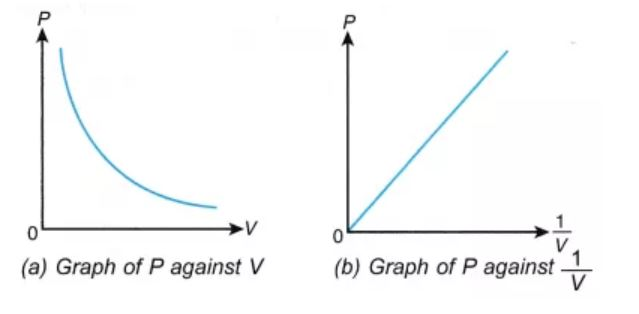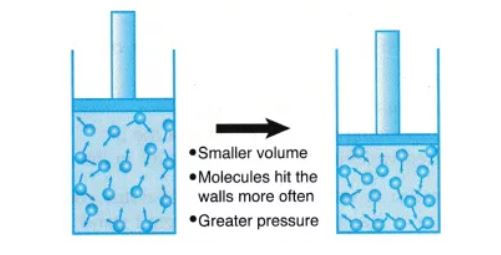# Boyle’s Law

## What is Boyle’s Law?

Boyle’s law is a gas law which states that the pressure exerted by a gas (of a given mass, kept at a constant temperature) is inversely proportional to the volume occupied by it. In other words, the pressure and volume of a gas are inversely proportional to each other as long as the temperature and the quantity of gas are kept constant. Boyle’s law was put forward by the Anglo-Irish chemist Robert Boyle in the year 1662.For a gas, the relationship between volume and pressure (at constant mass and temperature) can be expressed mathematically as follows.

P ∝ (1/V)

Where P is the pressure exerted by the gas and V is the volume occupied by it. This proportionality can be converted into an equation by adding a constant, k.

P = k*(1/V) ⇒ PV = k

The pressure v/s volume curve for a fixed amount of gas kept at constant temperature is illustrated below.It can be observed that a straight line is obtained when the pressure exerted by the gas (P) is taken on the Y-axis and the inverse of the volume occupied by the gas (1/V) is taken on the X-axis.

## Formula and Derivation

As per Boyle’s law, any change in the volume occupied by a gas (at constant quantity and temperature) will result in a change in the pressure exerted by it. In other words, the product of the initial pressure and the initial volume of a gas is equal to the product of its final pressure and final volume (at constant temperature and number of moles). This law can be expressed mathematically as follows:

P1V1 = P2V2

Where,

• P1 is the initial pressure exerted by the gas
• V1 is the initial volume occupied by the gas
• P2 is the final pressure exerted by the gas
• V2 is the final volume occupied by the gas

This expression can be obtained from the pressure-volume relationship suggested by Boyle’s law. For a fixed amount of gas kept at a constant temperature, PV = k. Therefore,

P1V1 = k (initial pressure * initial volume)

P2V2 = k (final pressure * final volume)

∴ P1V1 = P2V2

This equation can be used to predict the increase in the pressure exerted by a gas on the walls of its container when the volume of its container is decreased (and its quantity and absolute temperature remain unchanged).

## Examples of Boyle’s Law

When a filled balloon is squeezed, the volume occupied by the air inside the balloon decreases. This is accompanied by an increase in the pressure exerted by the air on the balloon, as a consequence of Boyle’s law. As the balloon is squeezed further, the increasing pressure eventually pops it. An illustration describing the increase in pressure that accompanies a decrease in the volume of a gas is provided below.If a scuba diver rapidly ascends from a deep zone towards the surface of the water, the decrease in the pressure can cause the gas molecules in his/her body to expand. These gas bubbles can go on to cause damage to the diver’s organs and can also result in death. This expansion of the gas caused by the ascension of the scuba diver is another example of Boyle’s law. Another similar example can be observed in the deep-sea fish that die after reaching the surface of the water (due to the expansion of dissolved gasses in their blood).

## Solved Exercises on Boyle’s Law

### Exercise 1

A fixed amount of a gas occupies a volume of 1L and exerts a pressure of 400 kPa on the walls of its container. What would be the pressure exerted by the gas if it is completely transferred into a new container having a volume of 3 litres (assuming the temperature and quantity of gas remains constant)?

Given,

Initial volume (V1) = 1L

Initial pressure (P1) = 400 kPa

Final volume (V2) = 3L

As per Boyle’s law, P1V1 = P2V2 ⇒ P2 = (P1V1)/V2

P2 = (1L * 400 kPa)/3L = 133.33 kPa

Therefore, the gas exerts a pressure of 133.33 kPa on the walls of the 3 liter container.

### Exercise 2

A gas exerts a pressure of 3 kPa on the walls of container 1. When container 1 is emptied into a 10-litre container, the pressure exerted by the gas increases to 6 kPa. Find the volume of container 1. Assume that the temperature and quantity of the gas remain constant.

Given,

Initial pressure, P1 = 3kPa

Final pressure, P2 = 6kPa

Final volume, V2 = 10L

According to Boyle’s law, V1 = (P2V2)/P1

V1 = (6 kPa * 10L)/3kPa = 20L

Therefore, the volume of container 1 is 20L.